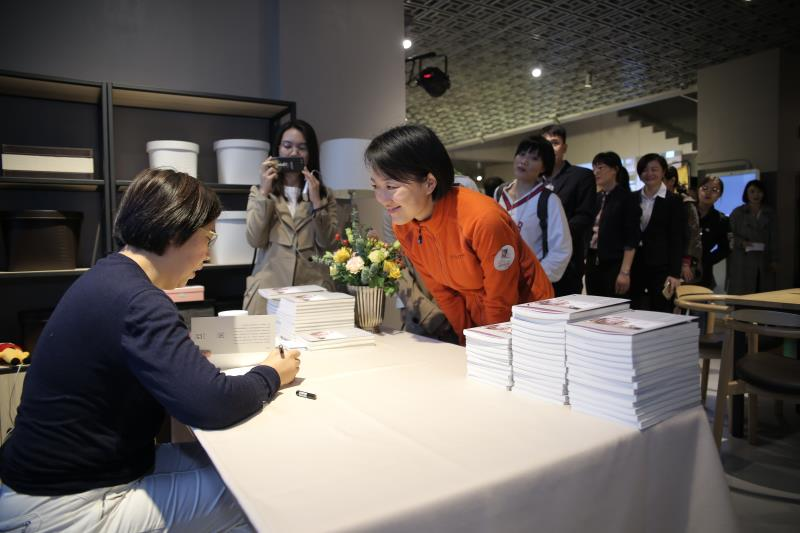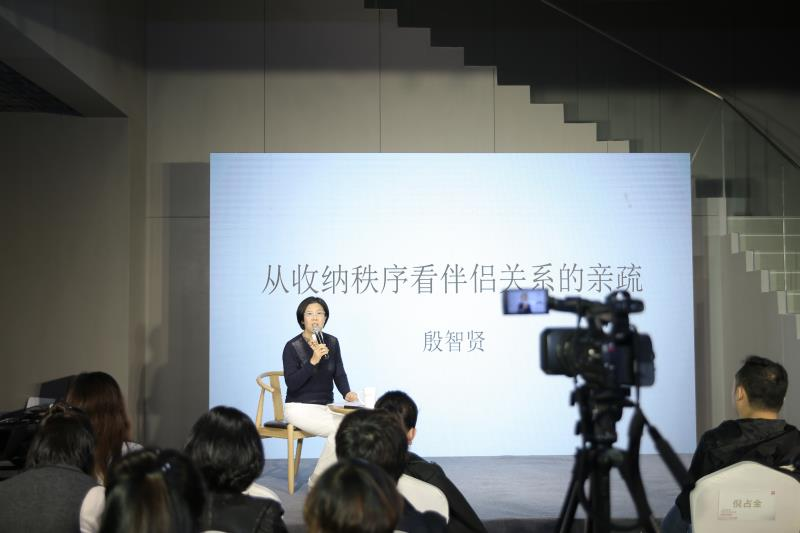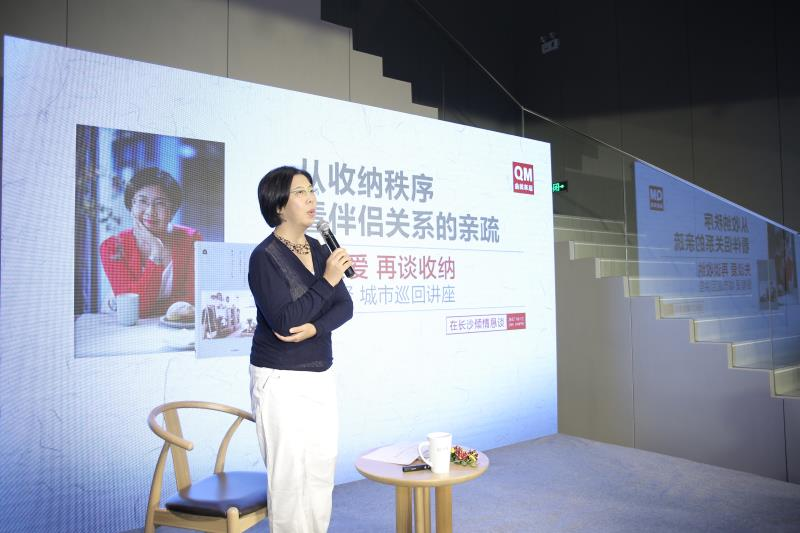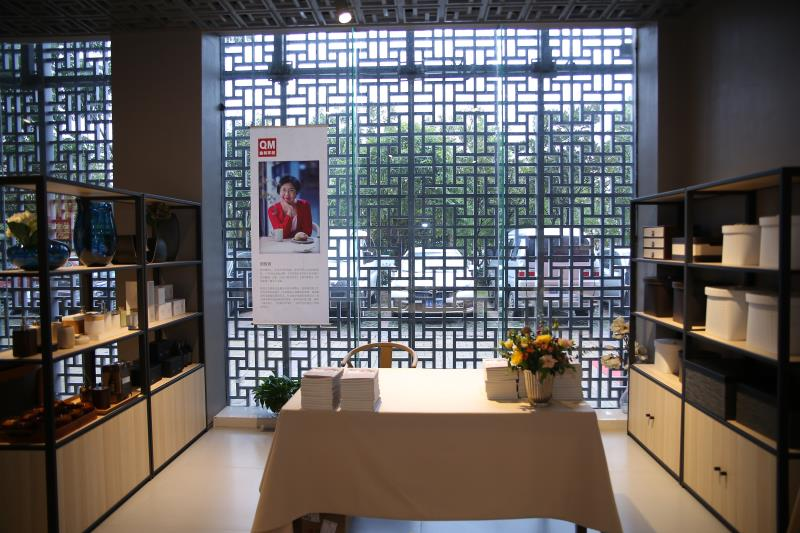|

# 你和Ta够亲密吗?一起干一场家务活看看

《圣经》说，上帝是用亚当的一根肋骨造出了夏娃；《诗经》曰，关关雎鸠，在河之洲。窈窕淑女，君子好逑；唐朝女诗人李冶《八至》里写到，至近至远东西，至深至浅清溪。至高至明日月，至亲至疏夫妻；《后汉书 梁鸿传》里讲，“为人赁舂，每归，妻为具食，不敢于鸿前仰视，举案齐眉。

鸳鸯双栖双飞，狼一生只选择一个爱人，天鹅总是出双入对。放眼大自然，伴侣从来都是一种不可或缺的关系。而人类更是如此，找一个人相爱到老、和一个人共度此生、陪一个人走到天涯海角……通过亲密的关系，每一个人，都得以获取精神的滋养，也能得到苍茫世界里坚强生存的动力。

或许，人总是认为自己不够完整，因此，一个伴侣被当作寄托，来实现一个完整的人生。

10月12日，由曲美家居主办的“先谈爱再谈收纳”暨《整理家整理亲密关系》作者殷智贤巡回讲座在长沙精彩上演。作为生活方式的提供者，曲美早已不再是一家简单的家具店，通过聚焦家庭亲密关系的建立，曲美希望传递“营造亲密关系引领城市美学”的理念，让生活更有温度。人类最需要的是亲密关系

在资深媒体人、生活方式研究者殷智贤看来，伴侣关系的亲疏在中国古代是有范本的，无论是举案齐眉，还是相敬如宾，那是全社会都达成共识的标准。然而在如今，标准失范了，大家都各有标准，每个人对待伴侣关系的评判标准都有所不同。

的确，为什么当下社会离婚率高居不下？为什么许多伴侣把日子过成了戏？为什么一段关系里的人往往缺乏安全感？为什么出轨、财产纠纷的事情在电视里一遍遍上演？为什么许多时候都不能理解枕边人？为什么身家丰厚的有钱人总是容易抑郁和不满足？

在殷智贤的新书里，看似亲切简单的背后，其实藏着很多深刻的关于社会学的思考和洞察。殷智贤表示，许多问题的答案或许就藏在对待家的态度上，生活中的很多微小细节往往正是反应伴侣关系的试金石，而人的生活方式更是被社会关系所影响着。殷智贤说，人的本能就是需要亲密关系，可许多人在对幸福、长久、安全、可靠的关系上却抱有很大的不确定性，这就是因为当前的社会环境让很多伴侣没有安全感。亲密关系需要安全感来维系

提起长沙，首先让人想到的就是选秀、臭豆腐和满大街的酒吧，这个让人总和娱乐联系起来的城市，其实也是一座拥有悠久历史的文化名城。本次巡回讲座选择落地长沙，据说也是有原因的。据《长沙市城市总体规划（2003-2020年）》（2014年修订版）显示，长沙至2020年将建成为一个能承载千万级人口规模的大都市，城镇化水平81%。

殷智贤表示，城镇化水平的提升带来的最大表现就是城镇人口的急剧增长，长沙也将彻底由一个熟人社会转化为陌生人社会，而这个变化的后果就是加大了内心的不安全感。人一旦没有了安全感，一是什么无耻的事都敢做，因为没有人认识他，二是谁也不敢相信，而这也会造成整个社会建设的危机。其实，亲密关系的问题看似是两人之间的问题，但其实是一个更广层面的社会问题。

殷智贤对如何建立更好的亲密关系以及如何建立安全感也提出了一些建议。比如，相信无常，把每一天都当作最后一天来过，更愿意为对方去付出；比如，把伴侣关系当成医患关系，大多数人都有心理创伤，不要要求对方做那些做不到的事，结婚后的许多矛盾就是由对方的创伤造成的；比如，用教练关系来看待伴侣，由于许多人心智不成熟，要知道如何教他；比如，修行，把两个人的关系当成一个修行。

殷智贤说，面对如今的困境，建立更有温度的亲密关系其实需要一些方法。一是，两个人在一起要有一个共同目标，当做任何决定的时候，要看有没有偏离这个目标；二是要有底线，要划出一些两个人都不能碰的线；三是注重细节，生活不提供轰轰烈烈的时间，大部分都是细水长流，把小事做好；四是沟通要成为习惯。

家庭环境微妙影响亲密关系

在本次活动上，通过对伴侣关系的解读，曲美试图用一种更具人性化的方式来诠释家庭环境和居家生活方式对亲密关系的影响。活动现场，还邀请到著名整理自媒体“第1整理术”出品人、日本专门家检定协会理事周一妍进行整理收纳知识分享。周一妍提出的居家整理观点是“你的家，就是你灵魂的模样”，她认为都市人追求简约生活，其实也是一种纾解压力，追求幸福感的方式。同时，她也提醒说，“断舍离”切勿过度，一切需要回归当下的自我，并且把家人关系摆放在“扔东西”的前面。伴随“先谈爱再谈收纳”城市巡回讲座的举办，曲美家居以自己的方式，再次展示出一个生活方式品牌养成背后所做的思考和投入，并输出为能够为用户带来具体成效的解决方案，成为一个真正用生活方式服务生活的品牌。

曲美相信，真正的生活方式品牌，不但要懂产品、懂设计、更要懂生活，而曲美正是秉持现代理念，运用科学的研究方法，吸纳中国传统文化和世界其他地区文化的精髓，力求为用户提供有效的生活问题解决方案，真正做到用好设计服务生活。

`声明：本文由入驻焦点开放平台的作者撰写，除焦点官方账号外，观点仅代表作者本人，不代表焦点立场错误信息举报电话： 400-099-0099，邮箱：jubao@vip.sohu.com，或点此进行意见反馈，或点此进行举报投诉。`A B C D E F G H J K L M N P Q R S T W X Y Z
A - B - C - D - E
• A
• 鞍山
• 安庆
• 安阳
• 安顺
• 安康
• 澳门
• B
• 北京
• 保定
• 包头
• 巴彦淖尔
• 本溪
• 蚌埠
• 亳州
• 滨州
• 北海
• 百色
• 巴中
• 毕节
• 保山
• 宝鸡
• 白银
• 巴州
• C
• 承德
• 沧州
• 长治
• 赤峰
• 朝阳
• 长春
• 常州
• 滁州
• 池州
• 长沙
• 常德
• 郴州
• 潮州
• 崇左
• 重庆
• 成都
• 楚雄
• 昌都
• 慈溪
• 常熟
• D
• 大同
• 大连
• 丹东
• 大庆
• 东营
• 德州
• 东莞
• 德阳
• 达州
• 大理
• 德宏
• 定西
• 儋州
• 东平
• E
• 鄂尔多斯
• 鄂州
• 恩施
F - G - H - I - J
• F
• 抚顺
• 阜新
• 阜阳
• 福州
• 抚州
• 佛山
• 防城港
• G
• 赣州
• 广州
• 桂林
• 贵港
• 广元
• 广安
• 贵阳
• 固原
• H
• 邯郸
• 衡水
• 呼和浩特
• 呼伦贝尔
• 葫芦岛
• 哈尔滨
• 黑河
• 淮安
• 杭州
• 湖州
• 合肥
• 淮南
• 淮北
• 黄山
• 菏泽
• 鹤壁
• 黄石
• 黄冈
• 衡阳
• 怀化
• 惠州
• 河源
• 贺州
• 河池
• 海口
• 红河
• 汉中
• 海东
• 怀来
• I
• J
• 晋中
• 锦州
• 吉林
• 鸡西
• 佳木斯
• 嘉兴
• 金华
• 景德镇
• 九江
• 吉安
• 济南
• 济宁
• 焦作
• 荆门
• 荆州
• 江门
• 揭阳
• 金昌
• 酒泉
• 嘉峪关
K - L - M - N - P
• K
• 开封
• 昆明
• 昆山
• L
• 廊坊
• 临汾
• 辽阳
• 连云港
• 丽水
• 六安
• 龙岩
• 莱芜
• 临沂
• 聊城
• 洛阳
• 漯河
• 娄底
• 柳州
• 来宾
• 泸州
• 乐山
• 六盘水
• 丽江
• 临沧
• 拉萨
• 林芝
• 兰州
• 陇南
• M
• 牡丹江
• 马鞍山
• 茂名
• 梅州
• 绵阳
• 眉山
• N
• 南京
• 南通
• 宁波
• 南平
• 宁德
• 南昌
• 南阳
• 南宁
• 内江
• 南充
• P
• 盘锦
• 莆田
• 平顶山
• 濮阳
• 攀枝花
• 普洱
• 平凉
Q - R - S - T - W
• Q
• 秦皇岛
• 齐齐哈尔
• 衢州
• 泉州
• 青岛
• 清远
• 钦州
• 黔南
• 曲靖
• 庆阳
• R
• 日照
• 日喀则
• S
• 石家庄
• 沈阳
• 双鸭山
• 绥化
• 上海
• 苏州
• 宿迁
• 绍兴
• 宿州
• 三明
• 上饶
• 三门峡
• 商丘
• 十堰
• 随州
• 邵阳
• 韶关
• 深圳
• 汕头
• 汕尾
• 三亚
• 三沙
• 遂宁
• 山南
• 商洛
• 石嘴山
• T
• 天津
• 唐山
• 太原
• 通辽
• 铁岭
• 泰州
• 台州
• 铜陵
• 泰安
• 铜仁
• 铜川
• 天水
• 天门
• W
• 乌海
• 乌兰察布
• 无锡
• 温州
• 芜湖
• 潍坊
• 威海
• 武汉
• 梧州
• 渭南
• 武威
• 吴忠
• 乌鲁木齐
X - Y - Z
• X
• 邢台
• 徐州
• 宣城
• 厦门
• 新乡
• 许昌
• 信阳
• 襄阳
• 孝感
• 咸宁
• 湘潭
• 湘西
• 西双版纳
• 西安
• 咸阳
• 西宁
• 仙桃
• 西昌
• Y
• 运城
• 营口
• 盐城
• 扬州
• 鹰潭
• 宜春
• 烟台
• 宜昌
• 岳阳
• 益阳
• 永州
• 阳江
• 云浮
• 玉林
• 宜宾
• 雅安
• 玉溪
• 延安
• 榆林
• 银川
• Z
• 张家口
• 镇江
• 舟山
• 漳州
• 淄博
• 枣庄
• 郑州
• 周口
• 驻马店
• 株洲
• 张家界
• 珠海
• 湛江
• 肇庆
• 中山
• 自贡
• 资阳
• 遵义
• 昭通
• 张掖
• 中卫

1室1厅1厨1卫1阳台

1
2
3
4
5

0
1
2

1

1

0
1
2
3报名成功，资料已提交审核A B C D E F G H J K L M N P Q R S T W X Y Z
A - B - C - D - E
• A
• 鞍山
• 安庆
• 安阳
• 安顺
• 安康
• 澳门
• B
• 北京
• 保定
• 包头
• 巴彦淖尔
• 本溪
• 蚌埠
• 亳州
• 滨州
• 北海
• 百色
• 巴中
• 毕节
• 保山
• 宝鸡
• 白银
• 巴州
• C
• 承德
• 沧州
• 长治
• 赤峰
• 朝阳
• 长春
• 常州
• 滁州
• 池州
• 长沙
• 常德
• 郴州
• 潮州
• 崇左
• 重庆
• 成都
• 楚雄
• 昌都
• 慈溪
• 常熟
• D
• 大同
• 大连
• 丹东
• 大庆
• 东营
• 德州
• 东莞
• 德阳
• 达州
• 大理
• 德宏
• 定西
• 儋州
• 东平
• E
• 鄂尔多斯
• 鄂州
• 恩施
F - G - H - I - J
• F
• 抚顺
• 阜新
• 阜阳
• 福州
• 抚州
• 佛山
• 防城港
• G
• 赣州
• 广州
• 桂林
• 贵港
• 广元
• 广安
• 贵阳
• 固原
• H
• 邯郸
• 衡水
• 呼和浩特
• 呼伦贝尔
• 葫芦岛
• 哈尔滨
• 黑河
• 淮安
• 杭州
• 湖州
• 合肥
• 淮南
• 淮北
• 黄山
• 菏泽
• 鹤壁
• 黄石
• 黄冈
• 衡阳
• 怀化
• 惠州
• 河源
• 贺州
• 河池
• 海口
• 红河
• 汉中
• 海东
• 怀来
• I
• J
• 晋中
• 锦州
• 吉林
• 鸡西
• 佳木斯
• 嘉兴
• 金华
• 景德镇
• 九江
• 吉安
• 济南
• 济宁
• 焦作
• 荆门
• 荆州
• 江门
• 揭阳
• 金昌
• 酒泉
• 嘉峪关
K - L - M - N - P
• K
• 开封
• 昆明
• 昆山
• L
• 廊坊
• 临汾
• 辽阳
• 连云港
• 丽水
• 六安
• 龙岩
• 莱芜
• 临沂
• 聊城
• 洛阳
• 漯河
• 娄底
• 柳州
• 来宾
• 泸州
• 乐山
• 六盘水
• 丽江
• 临沧
• 拉萨
• 林芝
• 兰州
• 陇南
• M
• 牡丹江
• 马鞍山
• 茂名
• 梅州
• 绵阳
• 眉山
• N
• 南京
• 南通
• 宁波
• 南平
• 宁德
• 南昌
• 南阳
• 南宁
• 内江
• 南充
• P
• 盘锦
• 莆田
• 平顶山
• 濮阳
• 攀枝花
• 普洱
• 平凉
Q - R - S - T - W
• Q
• 秦皇岛
• 齐齐哈尔
• 衢州
• 泉州
• 青岛
• 清远
• 钦州
• 黔南
• 曲靖
• 庆阳
• R
• 日照
• 日喀则
• S
• 石家庄
• 沈阳
• 双鸭山
• 绥化
• 上海
• 苏州
• 宿迁
• 绍兴
• 宿州
• 三明
• 上饶
• 三门峡
• 商丘
• 十堰
• 随州
• 邵阳
• 韶关
• 深圳
• 汕头
• 汕尾
• 三亚
• 三沙
• 遂宁
• 山南
• 商洛
• 石嘴山
• T
• 天津
• 唐山
• 太原
• 通辽
• 铁岭
• 泰州
• 台州
• 铜陵
• 泰安
• 铜仁
• 铜川
• 天水
• 天门
• W
• 乌海
• 乌兰察布
• 无锡
• 温州
• 芜湖
• 潍坊
• 威海
• 武汉
• 梧州
• 渭南
• 武威
• 吴忠
• 乌鲁木齐
X - Y - Z
• X
• 邢台
• 徐州
• 宣城
• 厦门
• 新乡
• 许昌
• 信阳
• 襄阳
• 孝感
• 咸宁
• 湘潭
• 湘西
• 西双版纳
• 西安
• 咸阳
• 西宁
• 仙桃
• 西昌
• Y
• 运城
• 营口
• 盐城
• 扬州
• 鹰潭
• 宜春
• 烟台
• 宜昌
• 岳阳
• 益阳
• 永州
• 阳江
• 云浮
• 玉林
• 宜宾
• 雅安
• 玉溪
• 延安
• 榆林
• 银川
• Z
• 张家口
• 镇江
• 舟山
• 漳州
• 淄博
• 枣庄
• 郑州
• 周口
• 驻马店
• 株洲
• 张家界
• 珠海
• 湛江
• 肇庆
• 中山
• 自贡
• 资阳
• 遵义
• 昭通
• 张掖
• 中卫• 手机• 分享
• 设计
免费设计
• 计算器
装修计算器
• 入驻
合作入驻
• 联系
联系我们
• 置顶
返回顶部